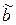CATEGORIES:

# Perforation of output sequences

Two components of turbo code encoder can be replaced by the code and change their relative information transfer rates. Different code rates R2, such as 1/2, 2/3, 3/4, etc. can be obtained from the code with a relative rate R1 = 1/3 through the perforations. For others relative information transfer rates of the encoder can contain more than two encode components.

Perforation is deletion of some elements from the output of the encoder to increase of the code rate.

For the codes with code rate R1 = 1/n we can obtain the rate R2 = k/np, where np = k*nl, using the matrix of perforations. The matrix of perforations is a matrix of size k*n containing l zeros. Zero indicates that the bit in the output sequence is not transmitted. The informative sequence a should be not perforated, i.e., zeros can not be in the first row of perforation matrix.

For example, R1 = 1/3 and it is required R2 = 2/5. So, n = 3, k = 2, np = 5. Let’s calculate l = 3*2 – 5 = 1. So, matrix of perforations can be:

 k columns n rows a bThe realization of perforation for the code combination of turbo code 010.110.111.011 will be 010.1X0.111.0X1. Note that for each two input bits the amount of output bits is 5, and the rate of the code is 2/5.

With the perforator, deleting of different number of the bits we can control the code rate. That is, we can construct encoder, adapting to the communication channel. For very noisy channels perforator deletes fewer bits, the code rate decreases and the noise immunity of encoder growth. If the communication channel of good quality, we can perforate a large number of bits, causing the information transfer rate growth.

Date: 2015-02-16; view: 1040

 <== previous page | next page ==> Turbo codes | Frames structure on HDLC procedure
doclecture.net - lectures - 2014-2023 year. Copyright infringement or personal data (0.006 sec.)